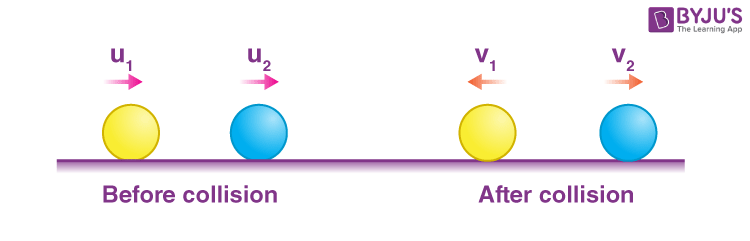# Elastic and Inelastic Collisions

We often hear in the news that two vehicles collided causing injuries to people. These collisions prove to be dangerous depending on the force they collide with each other. Let us try to find out how we can define collision? So to get started collision is a situation in which interacting bodies experience large forces for a short interval of time. The line along which the internal forces act during collision is known as the line of collision. Franck Hertz experiment explains about the elastic and inelastic collision.

## Types of Collision

Depending upon the velocity of the body with respect to the line of the collision, the collisions are classified into two types: Head-on Collision and Oblique Collision.

This type of collision happens when the velocities of both the particles are along the line of collision. This can be regarded as a collision in one dimension. A head-on collision is shown in the figure.### Oblique Collision

Oblique collision takes place when one of the two bodies has a velocity at an angle with the line of collision. In the case of an oblique collision, the component of velocity perpendicular to the line of the collision remains unchanged. This can be regarded as a collision in two dimensions.Oblique Collision

## To visualise what collisions actually are, click on the videos below## Elastic and Inelastic Collision

Apart from the above two classification collisions can also be classified on the basis of whether kinetic energy remains constant or not. If the kinetic energy of the system remains constant, then it is known as an elastic collision. While there are situations when some kinetic energy gets converted into heat, deformation of shape etc. These types of collisions are known as inelastic collisions.

## Coefficient of Restitution

We often define another quantity known coefficient of restitution (e). It is defined as the ratio of the velocity of separation by the velocity of approach along the line of collision. So here,

$$\begin{array}{l}e = \frac{v_2 – v_1}{u_1 – u_2}\end{array}$$Depending on the values of e we can infer the type of collision.

For e = 1, elastic collision

0 < e < 1, inelastic collision

e = 0, perfectly inelastic collision

## Watch the video and understand how airbags increase collision time during an impact## Frequently Asked Questions – FAQs

Q1

### What is elastic collision?

An elastic collision is a collision in which there is no net loss in kinetic energy in the system due to the collision.
Q2

### Is momentum conserved in the case of an inelastic collision?

Yes, momentum is conserved in an inelastic collision.
Q3

### What is an example of an elastic collision?

When you throw a ball on the ground, and it bounces back to your hand, there is no net change in the kinetic energy, and hence, it is an elastic collision.
Q4

### What is the difference between elastic and inelastic collision?

Unlike the elastic collision, where the kinetic energy is conserved, the kinetic energy in an inelastic collision is not conserved. In an inelastic collision, the kinetic energy between the colliding bodies is different at the beginning and the end of the collision.
Q5

### A car hitting a tree is an example of which type of collision?

It is an example of inelastic collision as the kinetic energy is not conserved here.